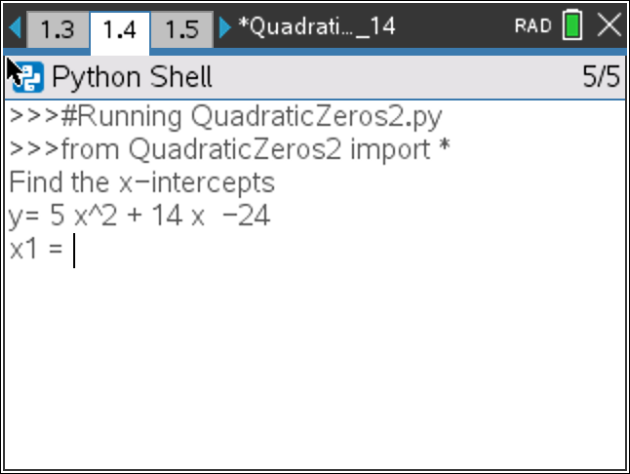# Activities

•6-8
9-12

50 Minutes

• ##### Device
•TI-Nspire™ CX II
•TI-Nspire™ CX II CAS
• ##### Software

TI-Nspire™ CX
TI-Nspire™ CX CAS

5.2

## Rational Quadratics Zeros#### Activity Overview

In this coding activity, students will extend the code from Integer Quadratic Zeros to create a game that allows exploration of other factored equation forms.

#### Objectives

• Use the input function and a variable to collect and store data from a user
• Use the randint() function to generate random integers.
• Use a while loop to repeat code
• Use if..elif..else statements to make decisions.
• Explore how x-intercepts are related to factored quadratic equations
• Explore how to factor equation in standard form
• Factor quadratic equations with rational solutions

#### About the Lesson

In this lesson, students will extend the code from Integer Quadratic Zeros.  If students didn’t complete the activity, complete that activity first or be sure to distribute base code to your students (TNS 1_Base Code).

In this lesson, you will create a game that lets you practice finding x-intercepts for equations in the form
y = ax2 + bx + c.  These solutions will have one rational and one integer solution.

In the challenge, you will apply what you have learned to create a third game.  This game will let you practice finding x-intercepts for equations in the form
y = ax2 + bx + c where both x-intercepts are rational numbers.

Note: This lesson requires the use of TI-Nspire™ CX II technology with OS 5.2 and above.